# TPC Decoder

Turbo product code (TPC) decoder

• Library:
• Communications Toolbox / Error Detection and Correction / Block

•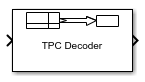## Description

The TPC Decoder block performs 2-D turbo product code (TPC) decoding of the soft input LLRs corresponding to the product code iteratively, using Chase-Pyndiah algorithm. The product code is a 2-D concatenation of linear block codes. The linear block code can be a parity check code, a Hamming code, or a BCH code capable of correcting two errors. Extended and shortened codes can be applied independently on each dimension. For a description of 2-D TPC decoding, see Algorithms.

For information about valid code pairs and the error-correcting capability for each valid code pair, see Component Code Pairs.

## Ports

### Input

expand all

Log likelihood ratios, specified as a column vector.

Data Types: `double` | `single`

### Output

expand all

TPC decoded message, returned as a column vector of binary values.

Data Types: `Boolean`

Actual number of decoding iterations, returned as a positive integer.

#### Dependencies

To enable this port, select Output number of iterations executed.

Data Types: `double`

## Parameters

expand all

#### Row TPC parameters

Number of rows in the product code matrix, NR. The list of integer values varies depending on the setting for Extended codes.

Number of rows in the message matrix, KR. The list of integer values varies depending on the setting for Extended codes and Number of rows in code, Nr.

Select Specify shortened message length to specify a value for Number of rows in shortened message, Sr.

Number of rows in the shortened message matrix, SR, specified as an integer less than or equal to KR. When you specify this parameter, provide full-length NR and KR values to specify the (NR,KR) code pair. This code pair is then shortened to the (NRKR+SR,SR) code pair, where:

#### Dependencies

To enable this parameter, select Specify shortened message length.

Data Types: `double`

#### Column TPC parameters

Number of columns in the product code matrix, NC. The list of integer values varies depending on the setting for Extended codes.

Number of columns in the message matrix, KC. The list of integer values varies depending on the setting for Extended codes and Number of columns in code, Nc.

Select Specify shortened message length to specify a value for Number of columns in shortened message, Sc.

Number of columns in the shortened message matrix, SC, specified as an integer. When you specify this parameter, provide full-length NC and KC values to specify the (NC,KC) code pair. This code pair is then shortened to the (NCKC+SC, SC) code pair, where:

#### Dependencies

To enable this parameter, select Specify shortened message length.

Data Types: `double`

Maximum number of decoding iterations, specified as a positive integer.

Data Types: `double`

Select Stop iterating when code converges to terminate decoding early if the calculated syndrome or parity-check of the component code evaluates to zero before Maximum number of iterations.

Select this parameter to add the `Iter` output port and output the actual number of TPC decoding iterations performed.

Type of simulation to run, specified as `Code generation` or `Interpreted execution`.

• `Code generation` –– Simulate the model by using generated C code. The first time you run a simulation, Simulink® generates C code for the block. The C code is reused for subsequent simulations unless the model changes. This option requires additional startup time, but the speed of the subsequent simulations is faster than `Interpreted execution`.

• `Interpreted execution` –– Simulate the model by using the MATLAB® interpreter. This option requires less startup time than the `Code generation` method, but the speed of subsequent simulations is slower. In this mode, you can debug the source code of the block.

## Block Characteristics

 Data Types `Boolean` | `double` | `single` Multidimensional Signals `no` Variable-Size Signals `no`

expand all

## Algorithms

Turbo product codes (TPC) are a form of concatenated codes used as forward error correcting (FEC) codes. Two or more component block codes, such as systematic linear block codes, are used to construct TPCs. The TPC decoder achieves near-optimum decoding of product codes using Chase decoding and the Pyndiah algorithm to perform iterative soft input, soft output decoding. For a detailed description, see  and . This decoder implements an iterative soft input, soft output 2-D product code decoding, as described in , using two Linear Block Codes. The decoder expects the soft bit log likelihood ratios (LLRs) obtained from digital demodulation as the input signal.

TPC Decoding Full-Length Messages

TPC encoded full-length input messages are decoded using specified 2-D TPC code pairs. Row-wise decoding uses the (NC,KC) code pair and column-wise decoding uses the (NR,KR) code pair. The input vector length must be NR × NC. To perform the 2-D TPC decoding, the column vector of the input LLRs, composed of the message and parity bits, is arranged into an NR-by-NC matrix.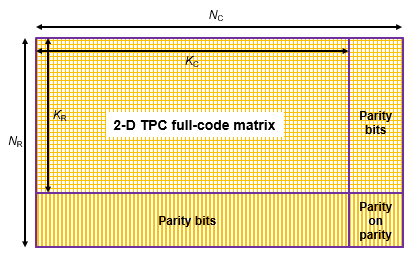The TPC decoder achieves near-optimum decoding of product codes using Chase decoding and the Pyndiah algorithm to perform iterative soft input, soft output decoding. Chase decoding forms a set of possible codewords for each row or column. The Pyndiah algorithm calculates soft information required for the next decoding step.

Iterative Soft Input, Soft Output Decoder

The iterative soft input, soft output decoding, as shown in the block diagram, carries out two decoding steps for each iteration.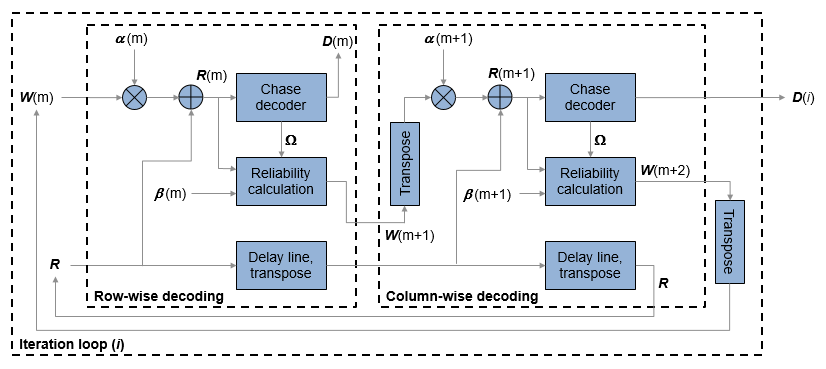The soft inputs for decoding are R(m) = R + α(m)W(m).

• Iteration loop counter i increments from i = 1 to the specified number of iterations.

• m = `2`i`1` is the decoding step index.

• R is the received LLR matrix.

• R(m) is the soft input for the mth decoding step.

• W(m) is the input extrinsic information for the mth decoding step.

• α(m) = [0,0.2,0.3,0.5,0.7,0.9,1,1, ...], where α is a weighting factor applied based on the decoding step index. For higher decoding steps, α = 1.

• β(m) = [0.2,0.4,0.6,0.8,1,1, ...], where β is a reliability factor applied based on the decoding step index. For higher decoding steps, β = 1.

• D contains the decoded message bits. The output message bits are formed from D by mapping –1 to 0 and +1 to 1, then reshaping the message block into a column vector.

The output message bits are formed after iterating through the specified number of iterations, or, if early termination is enabled, after code convergence.

Early Termination of TPC Decoding

If early termination is enabled, a code convergence check is performed on the hard decision of the soft input in each row-wise and column-wise decoding step. Early termination can be triggered after either the row-wise decoding or column-wise decoding converges.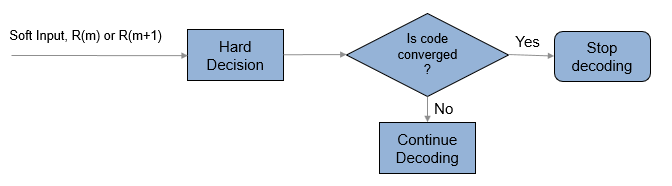The code is converged if, for all rows or all columns,

• The syndrome evaluates to zero in the codes (Hamming codes, Extended Hamming codes, BCH codes, or Extended BCH codes).

• The parity check is evaluated to zero in parity check codes.

The reported number of iterations evaluates to the iteration value that is currently in progress. For example, if the code convergence check is satisfied after row-wise decoding in the third iteration (after 2.5 decoding steps), then the number of iteration returned is 3.

TPC Decoding Shortened Messages

TPC encoded shortened input messages are decoded using specified 2-D TPC code pairs. Row-wise decoding uses the (NCKC + SC, SC) code pair and column-wise decoding uses the (NRKR + SR, SR) code pair. The input vector length must be (NRKR + SR) × (NCKC + SC). To perform the 2-D TPC decoding of shortened messages, the column vector of the input LLRs, composed of the shortened message and parity bits, is arranged into an (NRKR + SR)-by-(NCKC + SC) matrix.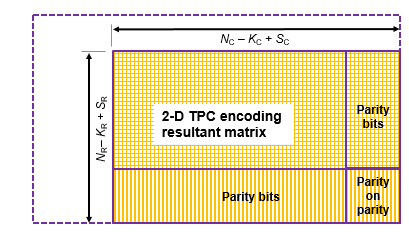The TPC decoder processes the received shortened message LLRs similar to full length codes, with these exceptions:

• The shortened bit positions in the received codeword are set to –1.

• The Chase algorithm does not consider the shortened bit positions while choosing the least reliable bits.

 Chase, D. "Class of Algorithms for Decoding Block Codes with Channel Measurement Information." IEEE Transactions on Information Theory, Volume 18, Number 1, January 1972, pp. 170–182.

 Pyndiah, R. M. "Near-Optimum Decoding of Product Codes: Block Turbo Codes." IEEE Transactions on Communications. Vol. 46, Number 8, August 1998, pp. 1003–1010.